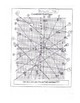# Graphing Linear Equation Review: Zombie Attack! Slope Intercept & Standard FormSubject
Resource Type
Product Rating
File Type

PDF (Acrobat) Document File

Be sure that you have an application to open this file type before downloading and/or purchasing.

1 MB|4 pages
Share
Product Description

This activity helps students review graphing linear equation that are written in both slope-intercept form and standard form. I created this worksheet this past year to try to make graphing equations more interesting. I didn't know at the time that there was already a similar worksheet on Tpt (actually, I didn't even know about Tpt at the time....). I think this worksheet has some distinct differences from the other one on here, although I haven't downloaded that one so am not sure as to the extent.

Students are given both sheets (don't copy them front to back - it is better if they are separate sheets, so they don't have to keep turning the paper over). Problems 1-7 are written in slope-intercept form, and can be graphed fairly easily. I tried to make a variety of problems - fraction slopes, whole # slopes, slope of -1 as well as some with a y-intercept and some without. I also included a zero slope equation and an undefined equation (my students always get those mixed up). The students graph the equations, and the line they graph must go through the head of a zombie to kill it (just like the TV show "The Walking Dead". They write the letter of the zombie they killed on the 1st sheet.

Problems 8-11 are written in standard form, and can be graphed using the x/y intercept method. I teach my students to try this method first if an equation is in standard form, as it is pretty easy to graph using the intercepts.

Problems 12-16 are also written in standard form, but they must be graphed by converting the equation to slope intercept. This is the toughest concept that my students have with graphing linear equations, but catch on with enough practice.

Most of my students completed this worksheet in our 53 minute period. I have included the answer key for this activity.

Total Pages
4 pages